The following article will visualize some mathematical models for brain cell activity. In some regions of the brain, neurons are excited or inhibited by neurons of a preceding input layer. They are called receptive field of that neuron. Since the visual area uses receptive fields as feature detectors (such as edge and edge-orientation detection) for natural images, the application of different receptive field functions on images can be nicely examined. The ipython notebook file to play with the parameters can be found on GitHub.

In :
import matplotlib.pyplot as plt
import matplotlib.image as mpimg
import scipy.signal as signal
import numpy as n


We examine the effect on the following images. In the visual pathway the images can be seen as input from the retina to higher visual areas.

In :
barImg=mpimg.imread('bar.png')
#extract grey values
barImg = barImg[:,:,3]

In :
imgplot = plt.imshow(barImg, cmap=cm.Greys_r)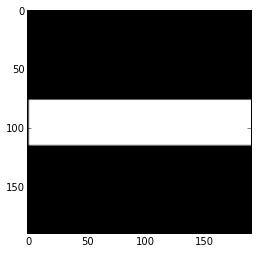In :
img=mpimg.imread('stinkbug.png')
#extract grey values
bugImg = img[:,:,0]

In :
imgplot = plt.imshow(bugImg, cmap=cm.Greys_r)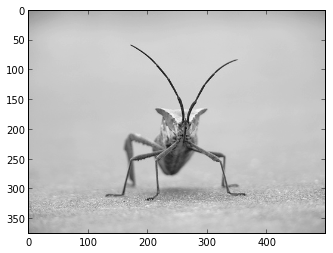## Receptive field functions¶

The two dimensional gaussian function is used in image processing as blurring filter. $$\phi(x,y) = \frac{1}{2\pi\sigma^2}\exp{(-\frac{1}{2\pi\sigma^2}(x^2+ y^2))}$$

In :
def gaussian2D(x, y, sigma):
return (1.0/(1*math.pi*(sigma**2)))*math.exp(-(1.0/(2*(sigma**2)))*(x**2 + y**2))


Since scipy's convolve function does not accept functions, we sample sample the function.

In :
"""make matrix from function"""
def receptiveFieldMatrix(func):
h = 30
g = zeros((h,h))
for xi in range(0,h):
for yi in range(0,h):
x = xi-h/2
y = yi-h/2
g[xi, yi] = func(x,y);
return g

In :
def plotFilter(fun):
g = receptiveFieldMatrix(fun)
plt.imshow(g, cmap=cm.Greys_r)


The gaussian function is circular symmetric, leading to excitation of a centered pixel from nearby pixels in convolution.

In the context of fourier transformation it is a low pass filter, which cancels out higher frequencies in the frequence domain of the image and is therefore blurring the image.

In :
plotFilter(lambda x,y:gaussian2D(x,y,4))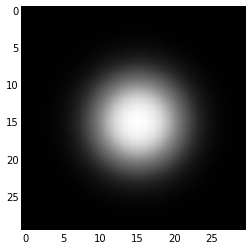Convolution is the process of applying the filter to the input, which is the image $I(x,y)$ denoting the grey value of the pixel at the specified position. $$\int \int I(x',y')\phi(x-x',y-y')dx'dy'$$

When applying the gaussian filter every neuron in the output layer is excited by nearby image neurons. The result of the convolution can then also be visualized in an image. Song Ho Ahn has a nice example for convolution of 2D Arrays.

In :
Img_barGaussian = signal.convolve(barImg,receptiveFieldMatrix(lambda x,y: gaussian2D(x,y,5)), mode='same')

In :
imgplot = plt.imshow(Img_barGaussian, cmap=cm.Greys_r)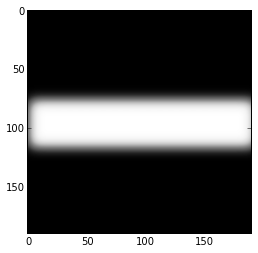In :
Img_bugGaussian = signal.convolve(bugImg,receptiveFieldMatrix(lambda x,y: gaussian2D(x,y,3)), mode='same')

In :
imgplot = plt.imshow(Img_bugGaussian, cmap=cm.Greys_r)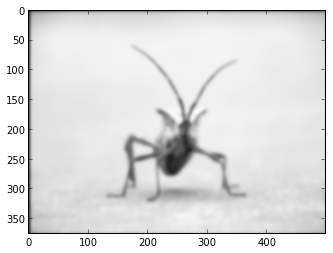## Difference of Gaussians¶

The mexican hat function is a difference of gaussians, which leads to an on-center, off-surround receptive field, found in retinal ganglion cells or LGN neurons. It can be seen as a basic edge detector.

In :
def mexicanHat(x,y,sigma1,sigma2):
return gaussian2D(x,y,sigma1) - gaussian2D(x,y,sigma2)

In :
plotFilter(lambda x,y: mexicanHat(x,y,3,4))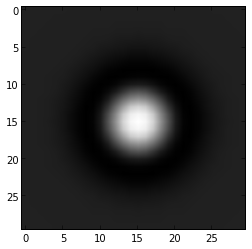In :
Img_barHat = signal.convolve(barImg,receptiveFieldMatrix(lambda x,y:mexicanHat(x,y,3,4)), mode='same')

In :
imgplot = plt.imshow(Img_barHat, cmap=cm.Greys_r)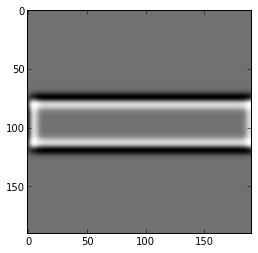In :
Img_bugHat = signal.convolve(bugImg,receptiveFieldMatrix(lambda x,y: mexicanHat(x,y,2,3)), mode='same')

In :
imgplot = plt.imshow(Img_bugHat, cmap=cm.Greys_r)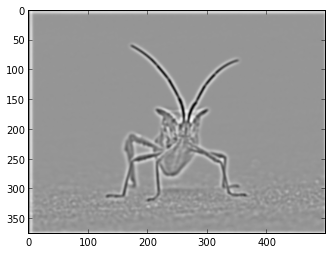## Gabor functions¶

Gabor functions are used to detect edges with a specific orientation in images. Neurons which can be modeled using gabor functions are found throughout the visual cortex.

Odd gabor: $$g_s(x,y):=sin(\omega_x x + \omega_y y)\exp{(-\frac{x^2+y^2}{2\sigma^2})}$$ Even gabor: $$g_c(x,y):=cos(\omega_x x + \omega_y y)\exp{(-\frac{x^2+y^2}{2\sigma^2})}$$

Orientation is given by the ratio $\omega_y/\omega_x$.

$g_s$ is activated by step edges, while $g_c$ is activated by line edges.

In :
def oddGabor2D(x,y,sigma,orientation):
return math.sin(x + orientation*y) * math.exp(-(x**2 + y**2)/(2*sigma))

In :
def evenGabor2D(x,y, sigma, orientation):
return math.cos(x + orientation*y) * math.exp(-(x**2 + y**2)/(2*sigma))

In :
plotFilter(lambda x,y: oddGabor2D(x,y,7,1))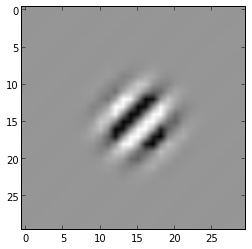In :
Img_barOddGabor = signal.convolve(barImg,receptiveFieldMatrix(lambda x,y: oddGabor2D(x,y,5,1)), mode='same')

In :
imgplot = plt.imshow(Img_barOddGabor, cmap=cm.Greys_r)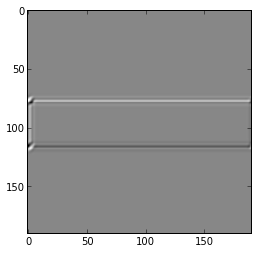In :
Img_bugOddGabor = signal.convolve(bugImg,receptiveFieldMatrix(lambda x,y: oddGabor2D(x,y,5,1)), mode='same')


In the following plot one can see clearly the edge orientations that excite the neuron.

In :
imgplot = plt.imshow(Img_bugOddGabor, cmap=cm.Greys_r)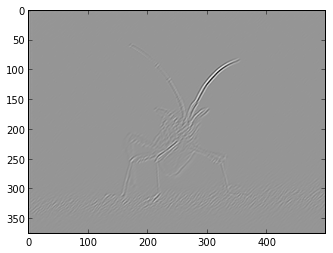Using the on-center, off-surround receptive field image as input to the gabor we obtain different results.

In :
Img_bugOddGaborEdge = signal.convolve(Img_bugHat,receptiveFieldMatrix(lambda x,y: oddGabor2D(x,y,5,1)), mode='same')

In :
imgplot = plt.imshow(Img_bugOddGaborEdge, cmap=cm.Greys_r)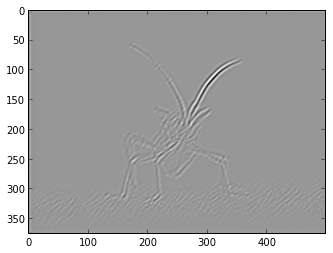In :
plotFilter(lambda x,y: evenGabor2D(x,y,7,1))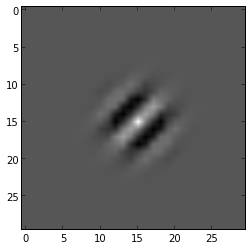In :
Img_barEvenGabor = signal.convolve(barImg,receptiveFieldMatrix(lambda x,y: evenGabor2D(x,y,5,1)), mode='same')

In :
imgplot = plt.imshow(Img_barEvenGabor, cmap=cm.Greys_r)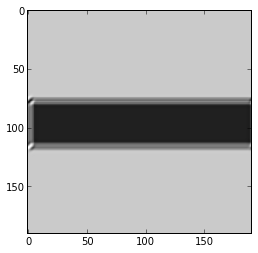In :
Img_bugEvenGabor = signal.convolve(bugImg,receptiveFieldMatrix(lambda x,y: evenGabor2D(x,y,5,1)), mode='same')

In :
imgplot = plt.imshow(Img_bugEvenGabor, cmap=cm.Greys_r)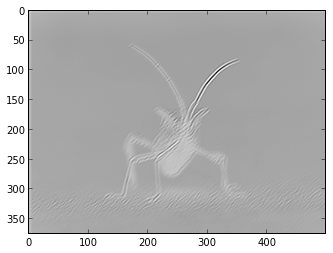A complex cell might react equally well to step edges and lines of either polarity. This is modeled by summing the squared responses of both odd and even gabor filter.

In :
def edgeEnergy(x,y,sigma, orientation):
g1= oddGabor2D(x,y,sigma,orientation)
g2= evenGabor2D(x,y,sigma,orientation)
return(g1**2+g2**2)

In :
plotFilter(lambda x,y:edgeEnergy(x,y,50,0))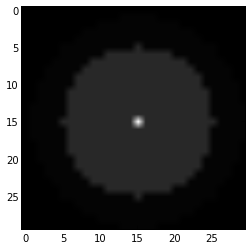In :
Img_barEdgeEnergy = signal.convolve(barImg,receptiveFieldMatrix(lambda x,y: edgeEnergy(x,y,100,1)), mode='same')
imgplot = plt.imshow(Img_barEdgeEnergy, cmap=cm.Greys_r)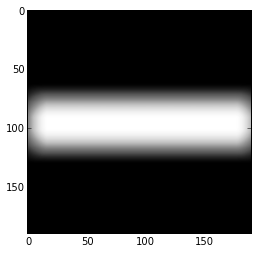In :
Img_bugEdgeEnergy = signal.convolve(bugImg,receptiveFieldMatrix(lambda x,y: edgeEnergy(x,y,10,1)), mode='same')
imgplot = plt.imshow(Img_bugEdgeEnergy, cmap=cm.Greys_r)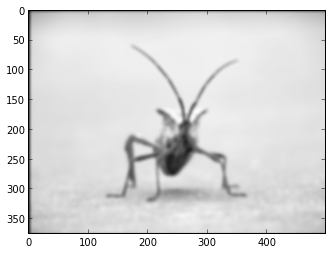## References ¶

Introduction to Computational Neuroscience, Hanspeter Mallot, University of Tübingen